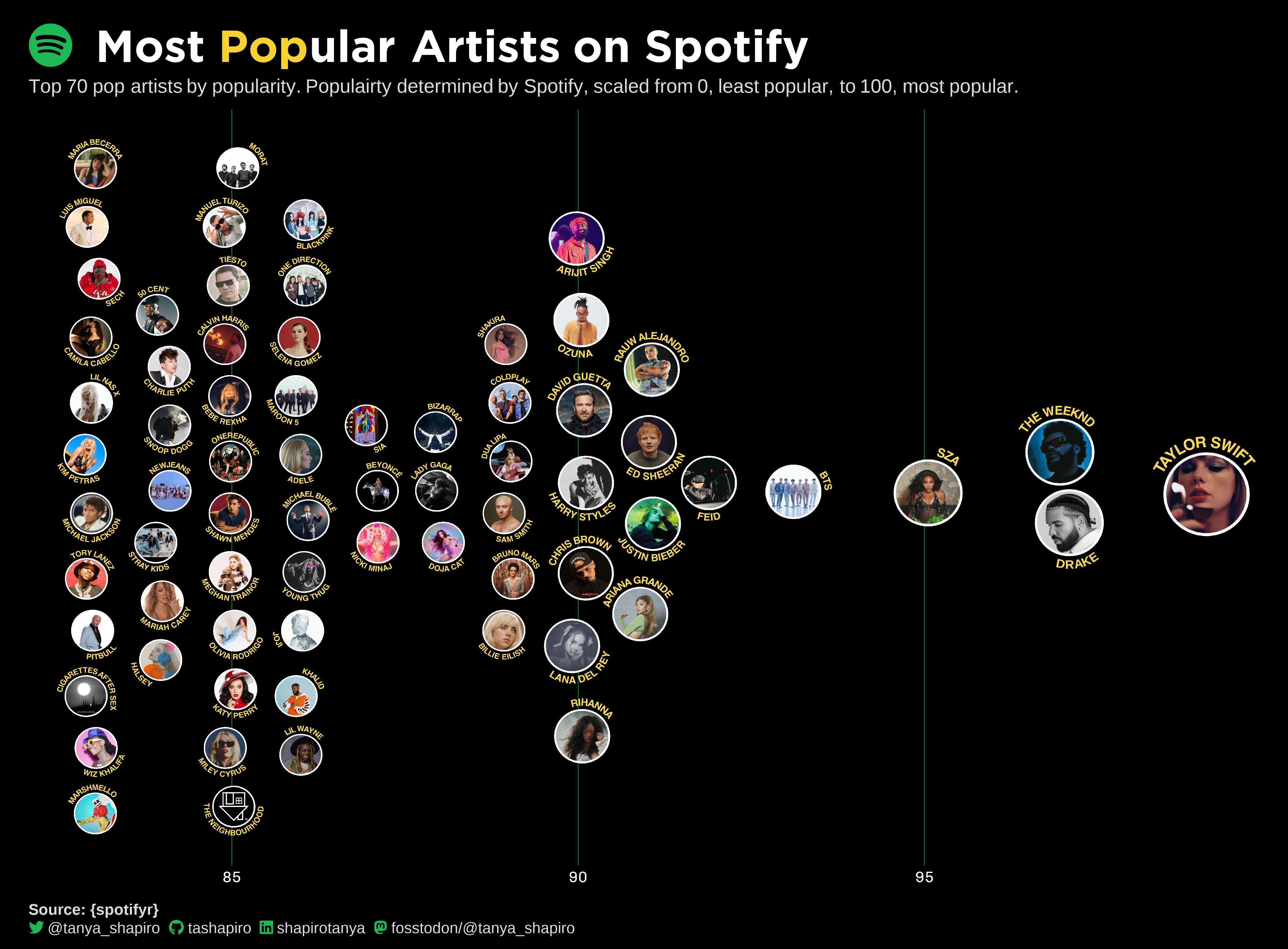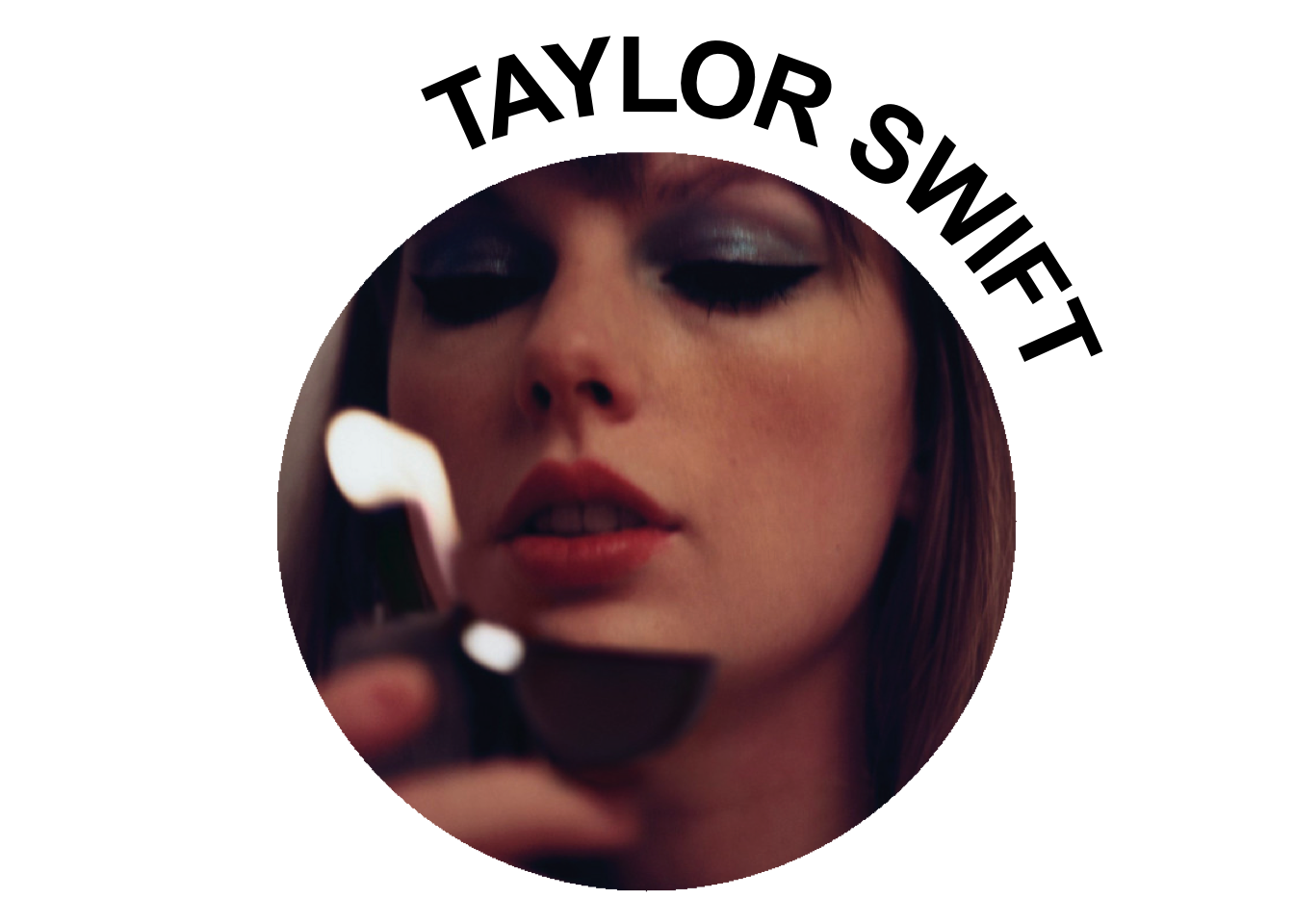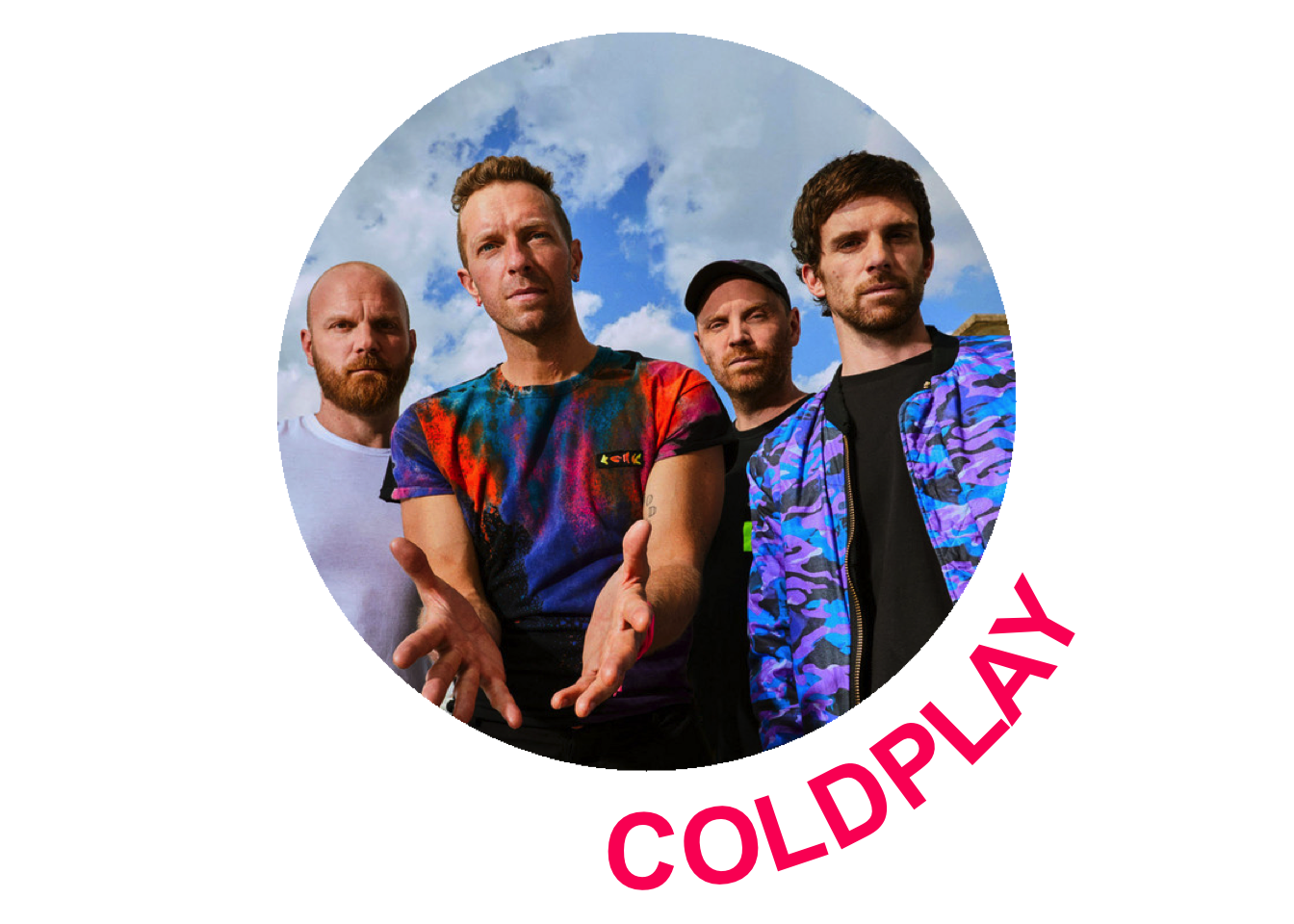# Wrapped Text Around Circular Images

Quick walk on setting up custom functions in R to create wrapped text around circular images.
R
ggplot2
image processing
Published

March 29, 2023

# Why on earth did I try this?

It’s no secret, I love working with images in data visualizations. When I’m working with `ggplot2`, I often import `ggimage` to plot images as geoms.

This idea of wrapping text around a circular image came about when I was experimenting with a beeswarm plot to show Spotify Artist’s popularity ratings. My idea was heavily inspired by one of my favorite pieces by Matt Daniels about hip-hop artists and their respective vocabulary sizes. But unlike Matt’s cool interactive graphic, my visual would remain static.

The problem I needed to solve for: how can I incorporate the artist’s name sans tooltip? How can we make the most use of space without overlapping? So I thought “well, let’s try text wrapping” - and that’s how this entire crazy experiment began.How I used text wrapping to generate my beeswarm plot of artists on Spotify

# Getting Started

After a lot of Googling and StackOverflowing, I discovered this was not going to be an easy walk in the park. No one package or library was going to solve my problem, I needed to do something custom.

Luckily, there were lots of cool libraries that addressed different parts of my problem. The heroes that did the heavy lifting include:

``````library(tidyverse)
library(geomtextpath)
library(ggimage)
library(cropcircles)
library(magick)
library(glue)``````

# Creating The Custom Function

Next step, we used these libraries to create our own custom function to generate a `ggplot2` rendering of the image with text.

The gist of the function: take the image, crop it into a circle with `cropcircles`, then use some math to create the outer curve for the text label that we’ll use with `geomtextpath`, and finally put it all together with `ggplot2`.

``````plot_image_label<-function(image,
label,
font_color="black",
position="top",
hjust=0.2){

#crop the image into a circle shape
cropped_image = cropcircles::circle_crop(image)

t = seq(0, 1, length.out = 100) * pi

#set up params based on top or bottom
if(position=="top"){
data = data.frame(x = cos(t),y = sin(t))
vjust=1.1
ymax=1.2
ymin=-0.9}

else if(position=="bottom"){
data=data.frame(x = cos(t),y = sin(t)*-1)
vjust=-0.1
ymax=0.9
ymin=-1.2}

#plot
ggplot() +
geom_image(aes(x=0, y=0, image = cropped_image), asp=2.4/2.1, size=.7) +
scale_x_continuous(limits = c(-1.2, 1.2))+
scale_y_continuous(limits=c(ymin, ymax))+
geom_textpath(data = data, aes(x,y,label =label), linecolor=NA, color=font_color,
size = 14.5,  fontface="bold", vjust = vjust, hjust=hjust)+
coord_equal()+
theme_void()
}``````

# Using The Function

Now let’s see it in action. For this example, I’m picking on TSwift.

``````#image of taylor swift
image_url<-"https://i.scdn.co/image/ab6761610000e5eb5a00969a4698c3132a15fbb0"

plot_image_label(image=image_url, label="TAYLOR SWIFT")``````Just for fun, let’s try another one. With the additional arguments, we can modify the text font and position (top or bottom).

``````#image of taylor swift
image_url_v2<-"https://i.scdn.co/image/ab6761610000e5eb989ed05e1f0570cc4726c2d3"

plot_image_label(image=image_url_v2, label="COLDPLAY", position="bottom", font_color="#FC1264")``````# Maximizing Functionality

Of course, my end game was to use these images for plotting. To plot them with ggplot2, I ended up saving these circle-text-wrap images in a new folder with `ggplot2::ggsave` (the power of the for loop can never be underestimated). After saving them, I then used their new directory paths to plug into `ggimage`. To see how I did this step, you can see the full code on my GitHub.

Thank you for following along, that’s a ~ wrap ~ !